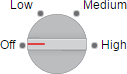# uiknob

## 语法

``kb = uiknob``
``kb = uiknob(style)``
``kb = uiknob(parent)``
``kb = uiknob(parent,style)``
``kb = uiknob(___,Name,Value)``

## 说明

``kb = uiknob` 在新图窗窗口中创建一个旋钮，并返回 `Knob` 对象。MATLAB® 调用 `uifigure` 函数来创建该图窗。`
``kb = uiknob(style)` 指定旋钮样式。`

``kb = uiknob(parent)` 在指定的父容器中创建旋钮。父容器可以是使用 `uifigure` 函数创建的 `Figure` 或其子容器之一。`

``kb = uiknob(parent,style)` 在指定的父容器中创建指定样式的旋钮。`

``kb = uiknob(___,Name,Value)` 使用一个或多个 `Name,Value` 对组参数指定对象属性。可将此选项与上述语法中的任何输入参数组合一起使用。`

## 示例

```fig = uifigure; kb = uiknob(fig);```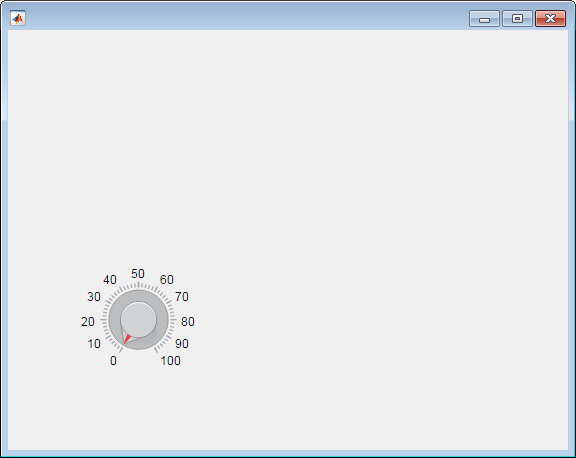```fig = uifigure('Position',[100 100 300 250]); kb = uiknob(fig,'discrete');```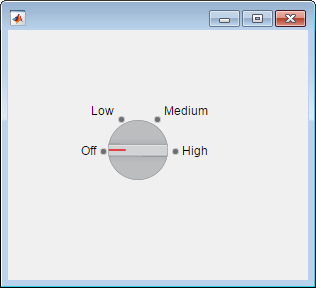```fig = uifigure; kb = uiknob(fig);```

```limits = kb.Limits ```
```limits = 0 100```

```kb.Limits = [-10 10]; kb.Value = 5;```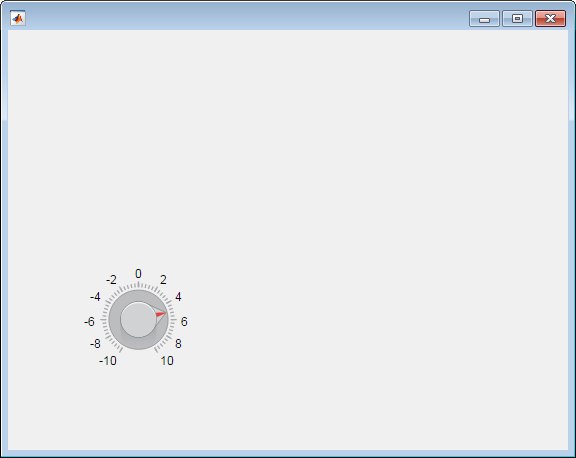```fig = uifigure; kb = uiknob(fig,'discrete'); ```

```kb.Items = {'Cold', 'Warm', 'Hot'}; kb.ItemsData = {32, 80, 212}; ```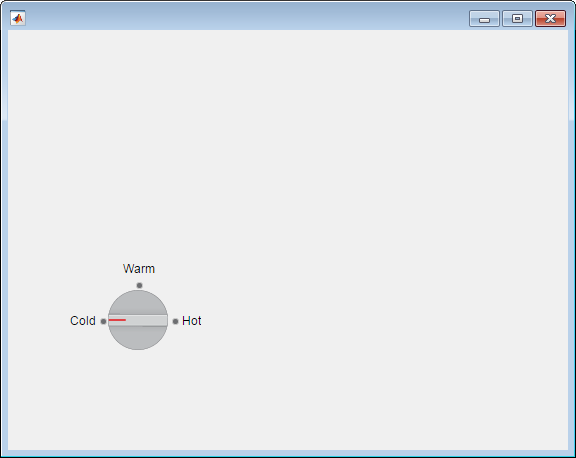`degrees = kb.Value`
```degrees = 32```

```function displayKnobValue % Create figure window fig = uifigure('Position',[100 100 283 275]); % Create the text field txt = uieditfield(fig,'text',... 'Position', [69 82 100 22]); % Create the knob kb = uiknob(fig,'discrete',... 'Position',[89 142 60 60],... 'ValueChangedFcn',@(kb,event) knobTurned(kb,txt)); end % Code the knob callback function function knobTurned(knob,txt) txt.Value = knob.Value; end```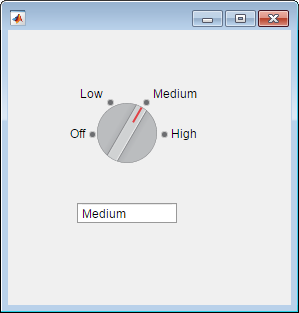```function showKnobValue % Create figure window and components fig = uifigure('Position',[100 100 283 275]); % Create label lbl = uilabel(fig,... 'Position',[218 177 50 15],... 'Text','0'); % Create knob kb = uiknob(fig,... 'Position',[89 142 60 60],... 'ValueChangedFcn', @(kb,event) knobTurned(kb,lbl)); end % Create ValueChangedFcn callback function knobTurned(kb,lbl) num = kb.Value; lbl.Text = num2str(num); end```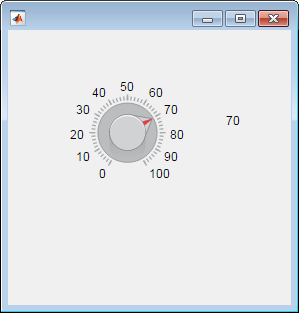```function showChangingValue % Create figure window fig = uifigure('Position',[100 100 283 275]); % Create numeric edit field num = uieditfield(fig,'numeric',... 'Position',[69 82 100 20]); % Create knob kb = uiknob(fig,... 'Position',[89 142 60 60],... 'ValueChangingFcn',@(kb,event) knobTurned(kb,event,num)); end % Create ValueChangingFcn callback function knobTurned(kb,event,num) num.Value = event.Value; end```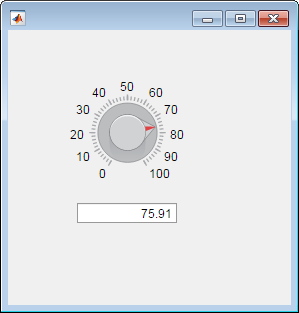```function increaseOnly % Create figure window fig = uifigure('Position',[100 100 400 275]); % Create knob kb = uiknob(fig,... 'Position',[150 125 60 60],... 'ValueChangedFcn',@(kb,event) nValChanged(kb,event,fig)); end % Create ValueChangedFcn callback function nValChanged(kb,event,fig) newvalue = event.Value; previousvalue = event.PreviousValue; if previousvalue > newvalue uialert(fig, 'Increase value only. Value reverted to previous value.', ... 'Invalid Value'); kb.Value = previousvalue; end end```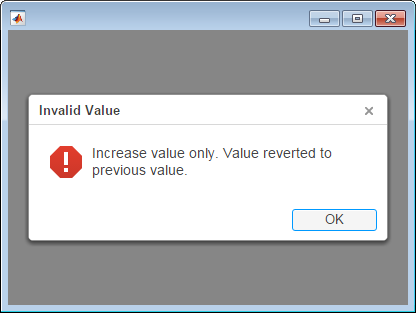## 输入参数

`'continuous'`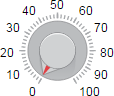`'discrete'`